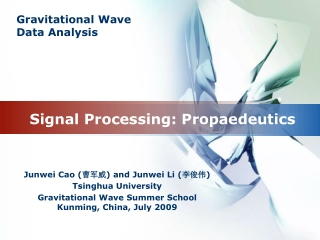DownloadDownload PresentationSignal Processing: Propaedeutics

# Signal Processing: Propaedeutics

Download Presentation## Signal Processing: Propaedeutics

- - - - - - - - - - - - - - - - - - - - - - - - - - - E N D - - - - - - - - - - - - - - - - - - - - - - - - - - -
##### Presentation Transcript

1. Signal Processing: Propaedeutics Junwei Cao (曹军威) and Junwei Li (李俊伟) Tsinghua University Gravitational Wave Summer School Kunming, China, July 2009

2. Signals • Signals: carriers in information transmitting • Deterministic signals • Random signals Deterministic Random

3. Time vs. Frequency

4. Unit Impulse • Dirac delta definition

5. Fourier Series • Periodic signal f(t) period: • Angular frequency: • Frequency:

6. Euler Formula • According to Euler formula • Rewrite Fourier series

7. Discrete vs. Continuous • When • periodic non-periodic • From discrete to continuous Discrete Continuous

8. Fourier Transformation • From Fourier series • Set

9. Fourier Inverse Transformation

10. An Example

11. An Exercise • A periodic cosine signal • Requirement: • Its Fourier transform • Draw the waveform of